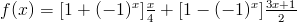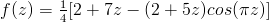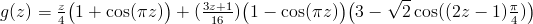### Archive

Posts Tagged ‘Fractals’

## The Collatz Conjecture as a Fractal

June 20th, 2009

The Collatz conjecture (or hailstone problem or 3n+1 problem) is a problem that is so simple to state that grade-schoolers can understand it, yet has been approached from an innumerable variety of angles and has resisted mathematicians for decades now. The problem is as follows:

Pick a positive integer. If it’s even then divide it by 2. If it’s odd, multiply it by 3 and add 1. Now apply this procedure to the result. Repeat. Will you always eventually hit 1 if you continue in this way?

For example, if you start with 11 then you multiply by 3 and add 1 to get 34, divide by 2 to get 17, and continuing similarly gets you the rest of the sequence: 52, 26, 13, 40, 20, 10, 5, 16, 8, 4, 2, 1. Before you go trying to find a counterexample to the conjecture, know that it has been verified by computer search for all starting numbers up to 20 × 258 ≈ 5.764 × 1018. The way that we are going to look at this problem today is the “pretty” approach; we’re going to extend the Collatz conjecture over the complex plane and look at the fractal defined by its iteration.

Define the Collatz function f(x) as follows:Take a moment or two to convinve yourself that if x is a positive integer, then f(x) is the next number after x in its Collatz sequence (e.g., f(11) = 34, f(34) = 17, and so on). To extend this function to the real numbers, simply recall that (-1)x = cos(πx). In fact, this gets us an extension to the complex numbers at the same time, and after some simplification we arrive at:Well hey, that’s a holomorphic function so it has a notion of a Julia set and we can study the fractal that its iterates induce. Indeed, we can obtain the following image pretty easily with standard fractal-generation software:

The fractal is located on the complex plane, and the horizontal line through the middle of the image is the real line. Black regions are regions in which the orbit of that number is bounded, while other colours indicate that the orbit of that number is unbounded (notice the large region of bounded numbers around z = 0). The big “spikes” that occur along the real line are, as we would expect, located at the integers (the image above is wide enough that you can see the spikes at z = -2, -1, 1, and 2). Instead of proceeding with the Collatz function as I have defined it, I’m going to introduce a modified Collatz function g(z) as follows:Observe that this function, like f(z), always maps natural numbers to terms that appear later in their Collatz sequence (e.g., g(11) = 17, g(17) = 13). The benefit of this function is that it has the additional property that g(1) = 1. That is, 1 is a fixed point of g(z), whereas it is part of a period-3 cycle of f(z). The fractal induced by g(z) is:

I originally moved to using g(z) instead of f(z) because the plot of the f(z) fractal from earlier indicated to me that there may be a ball of black (i.e., boundedness) of non-zero radius around each of the integers, but proving this for f(z) seemed to be quite difficult (as we would expect, since it would basically imply half of the Collatz conjecture). Somewhat strangely, even though there appear to be balls around the integers in the fractal induced by f(z), these balls vanish in the fractal induced by g(z). The image to the right is a close-up of the above fractal (the point of convergence is z = 1).

Nonetheless, the real line still seems to behave reasonably nicely under the action of g(z); it’s not difficult to prove that the fractal contains the real line segment [0,N] for some large number N that is similar in magnitude to the least M such that the conjecture is true for all n ≤ M (known to be at least 5×1018 or so, as mentioned earlier). However, many (non-natural number) points in that interval do not converge to 1.

So what now? If the Collatz conjecture is true, then z = 1 is the unique natural number fixed point of g(z), yet there seem to be points arbitrarily close to z = 1 that don’t even converge under iteration of g(z). Why are there smaller spikes between the integers in both of these fractals, and where are the spikes centered? Does the restriction of the Collatz function to the numbers at the center of those spikes have any simple interpretation? Who knows, I’ll explore some more in the future. Until then, enjoy some pretty pictures.

Tags: